Courses
Courses for Kids
Free study material
Free LIVE classes
More

# Velocity of Object and Velocity of Image for JEELIVE
Join Vedantu’s FREE Mastercalss

## Image Formation in Plane and Spherical Mirrors

In order to understand the difference in velocity between an object and its image on the mirror, it is important to understand the nature and geometry of the images formed on three major types of mirrors, namely plane mirror, concave mirror, and convex mirror. This plays an important role while we try to understand why the velocity of an image in a plane mirror is twice that of the object that produced that image and so on.

## Virtual and Real Images

The nature of the image can be effectively determined by three major criteria, which are as follows:

• Whether the image is real or virtual

• Whether the image size is reduced, same, or enlarged with respect to the object

• Whether the image is inverted or upright with respect to the object

Note: For real images, light passes through and diverges from the image. In simple terms, the image can be captured on a screen placed at the image location. However, for virtual images, light only diverges from the image and does not pass through it. Hence, virtual images cannot be captured on a screen.

## Plane Mirrors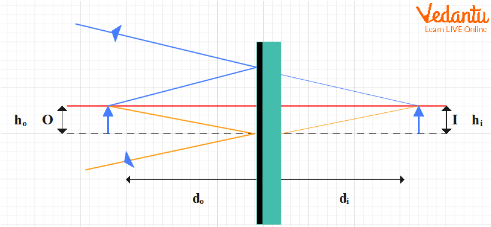Size and Position of Object and Image in a Plane Mirror

• The image in a plane mirror is at the same distance from the mirror as that between the mirror and the object.

• The size of the object and the image are the same.

• The magnification, i.e., the ratio of image and object height is equal to 1 for a plane mirror.

• The mirror equation for a plane mirror is u = -v, where v is the image distance, u is the object distance, and f is the focal length from the pole of the mirror.

## Spherical Mirrors (Concave and Convex)

• Both object and image are on the same side of the mirror.

• The nature and quality of the image formed in concave mirrors depend upon the curvature of the concave mirror and the relative location of the object with respect to the mirror.

• Likewise, for a convex mirror, the image and the object are on opposite sides of the mirror. The size of the object and the image are not the same.

• The mirror equation for a spherical mirror is $\frac{1}{v}+\frac{1}{u}=\frac{1}{f}$.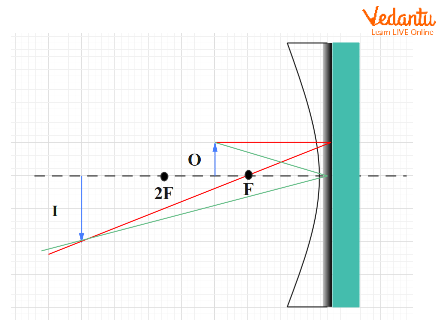Size and Position of Object and Image in a Concave Mirror

## Image and Object Velocity

An image is a reflection of any object on an optical element. In other words, the image is formed through convergence or divergence of rays of light emitted by the object on interaction with an optical element.

In some cases, the image and the object velocity might be the same, while in others they might be different. The relationship between image and object velocity depends on the state of rest or motion of the optical element with respect to the object, the position of the object, the type of optical element etc.

The mirror equations play a crucial role in finding the velocity of the image and object. The basic idea of the mirror equation is to establish a relationship between the distances of the image, object, and focus.

Let us now derive the relationship between the image and object velocity in a plane under various conditions and also velocity of image in a spherical mirror.

## Relation between Velocity of Object and Image in a Plane Mirror

### 1. When The Object is Located at The Centre of Curvature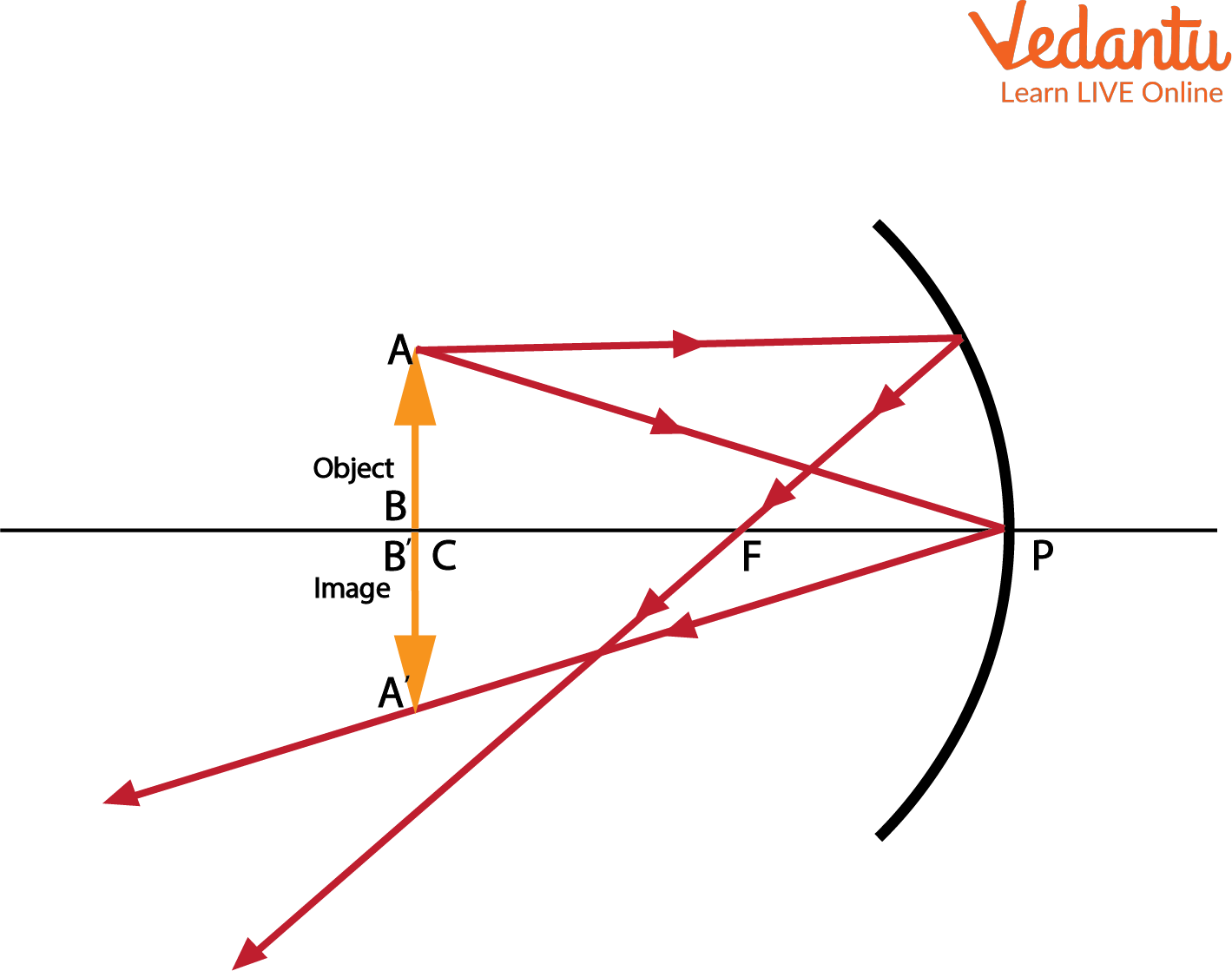Object AB is at the centre of the curvature of the mirror

By the nature of plane mirrors, it can be concluded that

$\frac{1}{v}+\frac{1}{u}=\frac{1}{f}$.

On differentiating the above equation with respect to time ‘t’,

$\frac{-1}{{{v}^{2}}}\frac{dv}{dt}-\frac{1}{{{u}^{2}}}\frac{du}{dt}=0$

Considering Vim is the velocity of the image with respect to the mirror and Vobj is the velocity of the object with respect to the mirror,

$\frac{-1}{{{v}^{2}}}{{V}_{im}}-\frac{1}{{{u}^{2}}}{{V}_{obj}}=0$

${{V}_{im}}=-\frac{{{v}^{2}}}{{{u}^{2}}}{{V}_{obj}}$

${{V}_{im}}=-{{m}^{2}}{{V}_{obj}}$

The negative sign indicates that the velocity of the image will increase if the velocity of the object decreases.

As $\left| \left. m \right| \right.=1\left| \left. \frac{dv}{dt} \right| \right.<\left| \left. -\frac{du}{dt} \right| \right.$

Hence, when the object is at the curvature centre,

$\left| \left. \frac{dv}{dt} \right| \right.=\left| \left. -\frac{du}{dt} \right| \right.$

### 2. When The Object Moves Between The Focus And The Centre of Curvature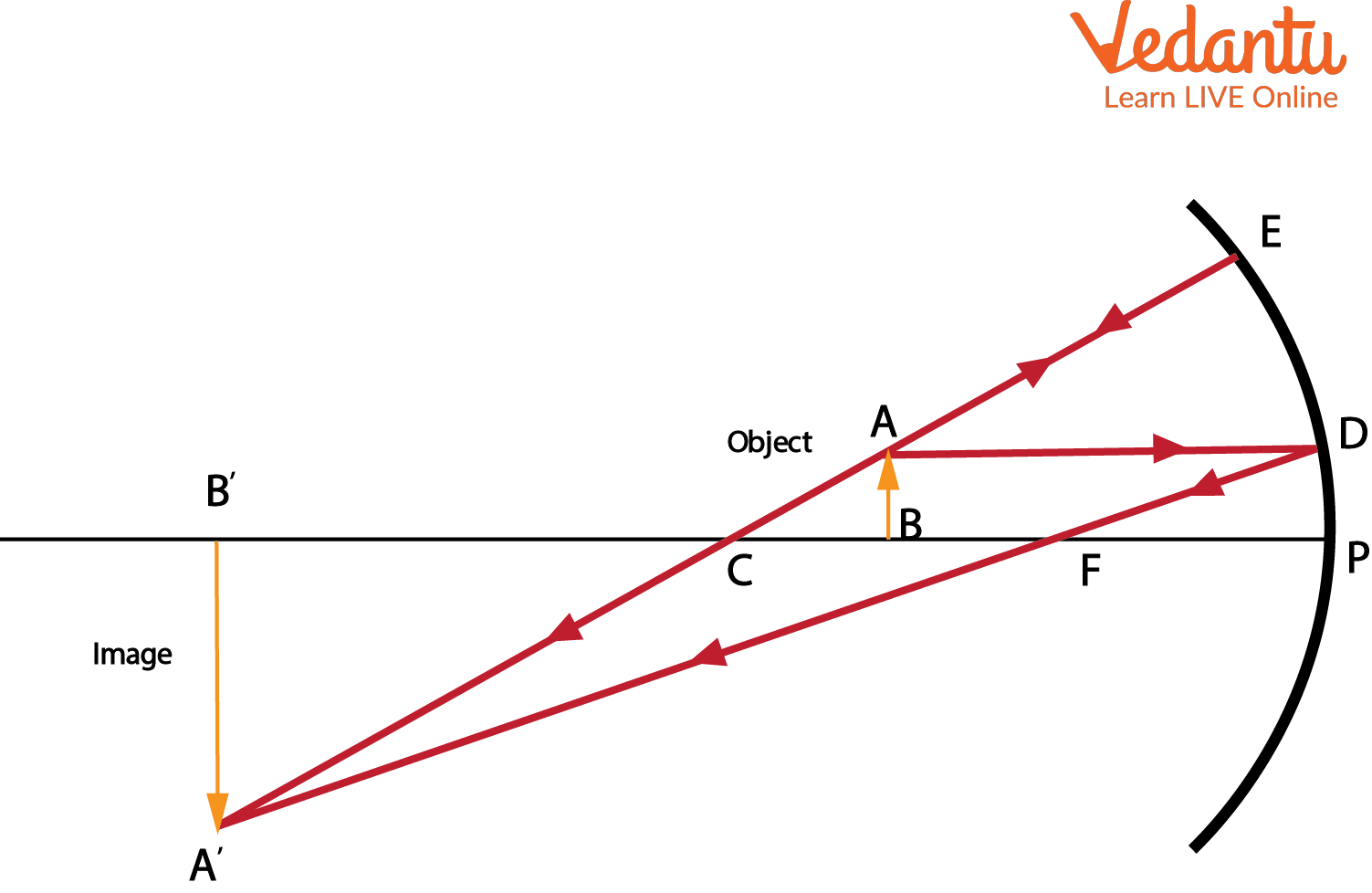Image: Object AB is between the centre of the curvature and the focus

$\left| \left. \frac{dv}{dt} \right| \right.>\left| \left. -\frac{du}{dt} \right| \right.$

### 3. When The Object Moves Between The Pole of The Mirror and The Focus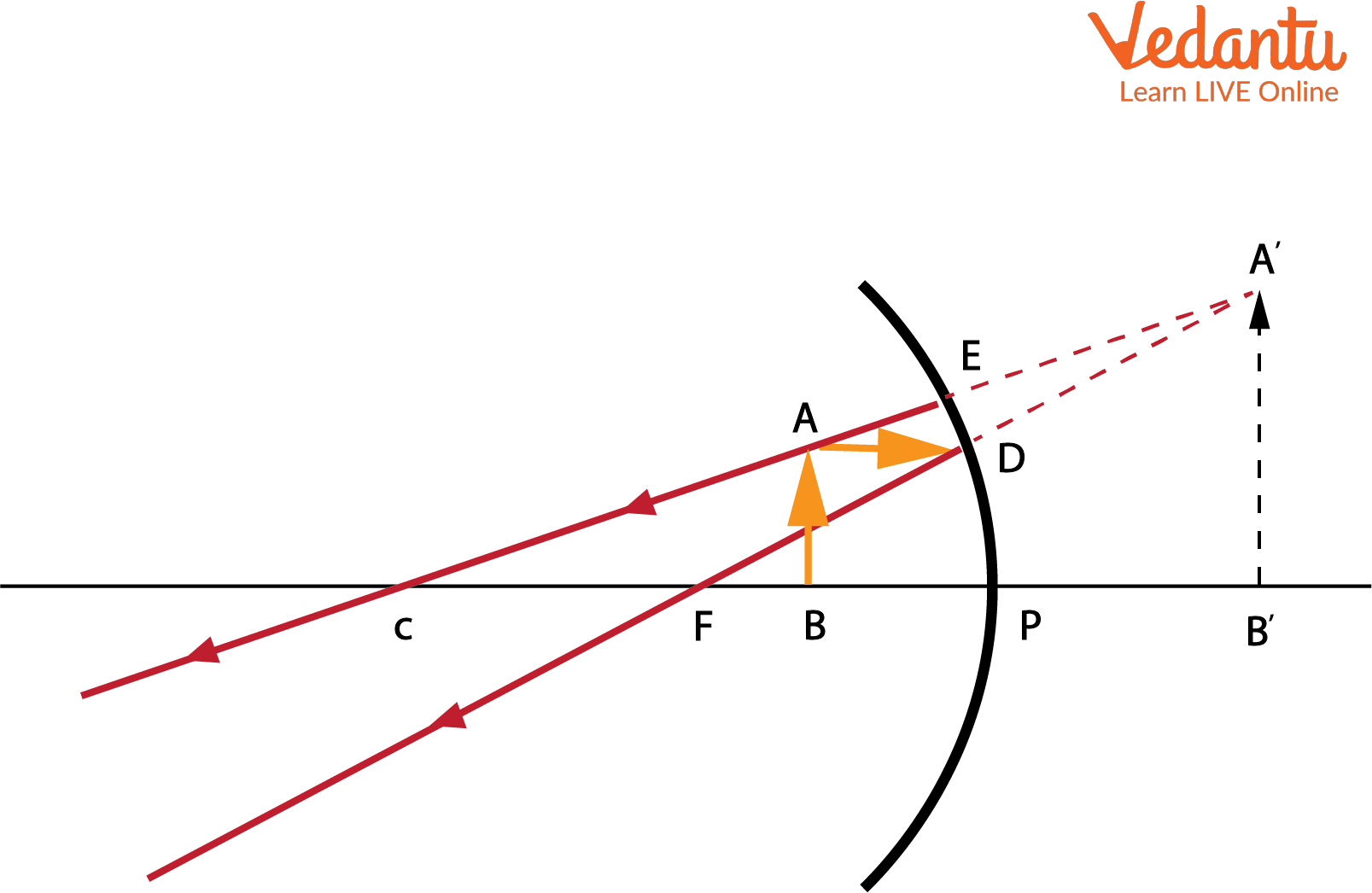Image: Object AB is between the Mirror and the Focus

As the image produced in such a case is virtual, the equation is given as

$\frac{1}{\left( +v \right)}+\frac{1}{\left( -u \right)}=\frac{1}{\left( -f \right)}$

Differentiating the above equation, we get

$\frac{dv}{dt}=\frac{{{v}^{2}}}{{{u}^{2}}}\frac{du}{dt}$

This signifies that if the object velocity decreases, the image velocity will also decrease. Here, $\left| \left. m \right| \right.>1$ hence the speed of the image will be more than that of the object. This is why side view mirrors in cars warn that the images might be closer than they appear.

Note: The velocities mentioned are with respect to the mirror, i.e., the object or image velocity with respect to ground and with respect to the mirror will be the same when the mirror is at rest. This is an important point to remember while solving JEE problems.

## Velocity of Image in Plane Mirror Formula

From the above cases, it can be concluded that the image velocity is the same as that of the object’s velocity in a plane mirror. In other words, when the object moves towards the mirror at a velocity of u m/s, the image also moves at a velocity of u m/s. Hence,

$\text{Velocit}{{\text{y}}_{\text{image}}}=\text{Velocit}{{\text{y}}_{\text{object}}}=u\text{ m/s}=\frac{\text{distance}}{\text{speed}}$

## Relative Velocity of the Image with respect to Object in a Plane Mirror

Since we know that

$\text{Velocit}{{\text{y}}_{\text{image}}}=\text{Velocit}{{\text{y}}_{\text{object}}}=u\text{ m/s}$

Distance between the object and the mirror = Distance between the image and the mirror = x

Distance between the object and the image $=x+x=2x$

Say an object covers a distance of u units in one second at a velocity of u m/s. Then the distance between the object and the mirror becomes,

Distance between the object and the mirror $=x-u$

The new distance between the object and the image $=\left( x-u \right)+\left( x-u \right)=2\left( x-u \right)$

The distance reduced between object and image in 1 second, is 2u.

Therefore, the relative velocity between the image and the object is 2u m/s as they are moving towards each other (in opposite direction).

## Important Concepts to Remember for JEE

• When an object moves in a perpendicular direction with respect to a plane mirror, either towards or away from the mirror with a speed u, the image also moves with the same speed u.

• However, in the above case, the relative speed of the image is 2u with respect to the object.

• When an object moves in an inclined manner with respect to the perpendicular plane of a plane mirror, with a speed v forming an angle 𝚹, the relative velocity of the object with respect to the image (the angle between the velocity vector of object and image) is given by:

$\overrightarrow{{{V}_{OI}}}=\overrightarrow{{{V}_{O}}}-\overrightarrow{{{V}_{I}}}=\left( V\cos \theta \hat{i}-V\sin \theta \hat{j} \right)+\left( V\cos \theta \hat{i}+V\sin \theta \hat{j} \right)=2V\cos \theta \hat{i}$

$\overrightarrow{{{V}_{OI}}}=\overrightarrow{{{V}_{O}}}-\overrightarrow{{{V}_{I}}}=2V\cos \theta$

## Summary

The velocities of the object and its image formed on the mirror will differ based on the nature and geometry of image formation. It is important to understand and calculate the relative velocity of objects and images formed on a plane and spherical mirrors as it helps in various applications.

Last updated date: 20th Sep 2023
Total views: 126.6k
Views today: 2.26k

## FAQs on Velocity of Object and Velocity of Image for JEE

1. Why does an image move with twice the object’s velocity in a plane mirror?

The distance between the object and the mirror and between the image formed and the mirror is the same, say x. But when considering the plane of the mirror as the y-axis, the object and the image lie on opposite sides of the x-axis. Hence the relative distance between the object and the image is 2x. An object moving towards the mirror with a speed u travels u units in 1 second. Now the distance between the object and the image is 2x - 2u. It can be seen that the distance is reduced by 2u in 1 second, confirming that the image travels at twice the object’s speed.

2. What are the important sign conventions in a spherical mirror problem? Why is it important?

Sign conventions are very important because they capture the actual and relative values of the elements involved, thereby characterising the optical system. Also, the sign conventions lead to a better understanding of images and objects even without the help of a ray diagram. The following are the most important sign conventions that will help you out during the exams:

• For concave mirrors, the focal length is positive, whereas, for convex mirrors, it is negative.

• The distance of the image is positive with respect to the pole of the mirror for images that are real and negative for images that are virtual.